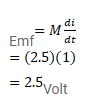# The mutual inductance between two coils isQuestion:

The mutual inductance between two coils is $2.5 \mathrm{H}$. If the current in one coil is changed at the rate of $1 \mathrm{~A} / \mathrm{s}$, what will be the emf induced in the other coil?

Solution: